# 什麼是字典樹

### 什麼是字典樹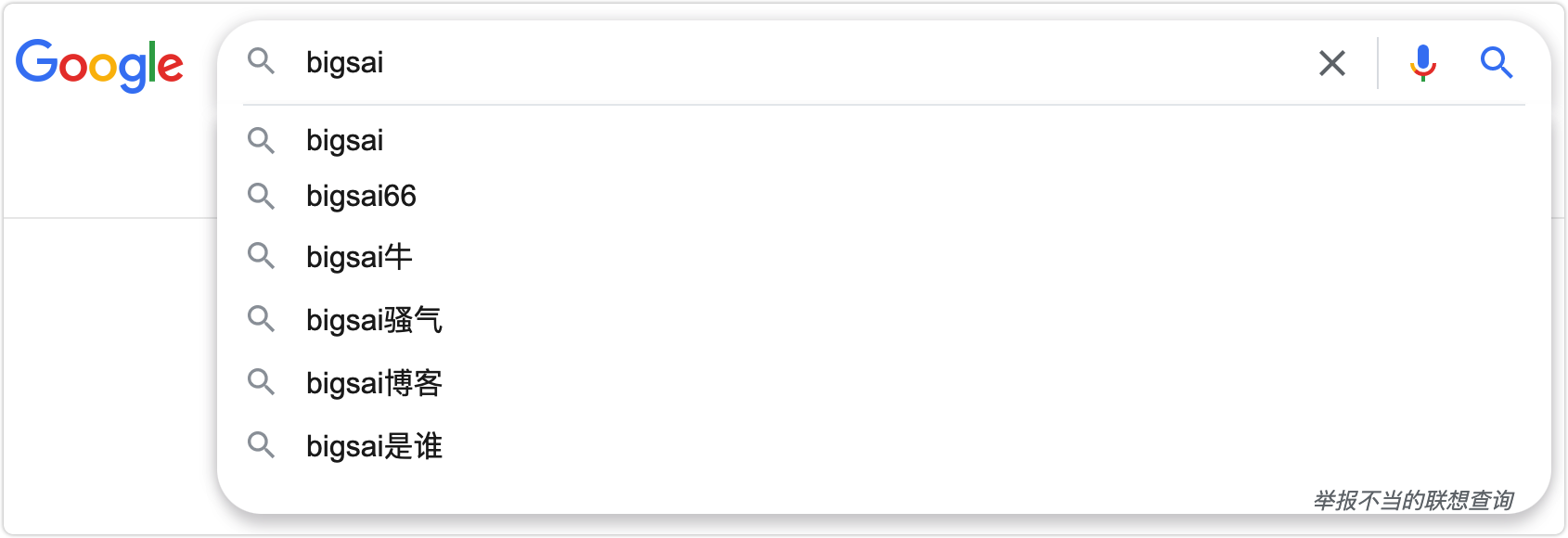1：根節點不包含字元，除了根節點每個節點都只包含一個字元。root節點不含字元這樣做的目的是為了能夠包括所有字串。

2：從根節點到某一個節點，路過字串起來就是該節點對應的字串。

3：每個節點的子節點字元不同，也就是找到對應單詞、字元是唯一的。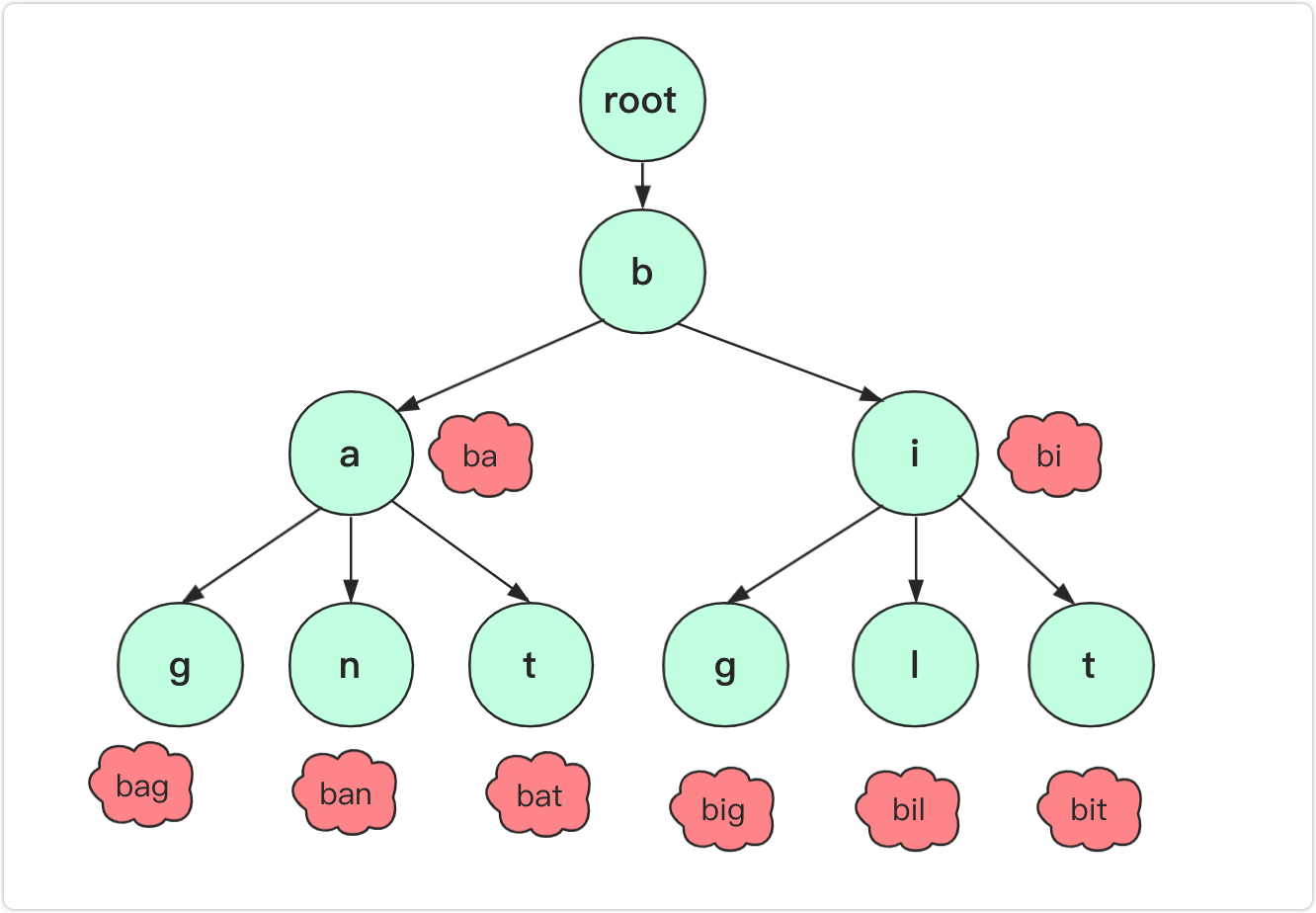### 設計實現字典樹

• Trie() 初始化字首樹物件。
• void insert(String word) 向前綴樹中插入字串 word 。
• boolean search(String word) 如果字串 word 在字首樹中，返回 true（即，在檢索之前已經插入）；否則，返回 false 。
• boolean startsWith(String prefix) 如果之前已經插入的字串 word 的字首之一為 prefix ，返回 true ；否則，返回 false 。

```java class TrieNode { TrieNode son[]; boolean isEnd;//結束標誌 public TrieNode()//初始化 { son=new TrieNode; } }```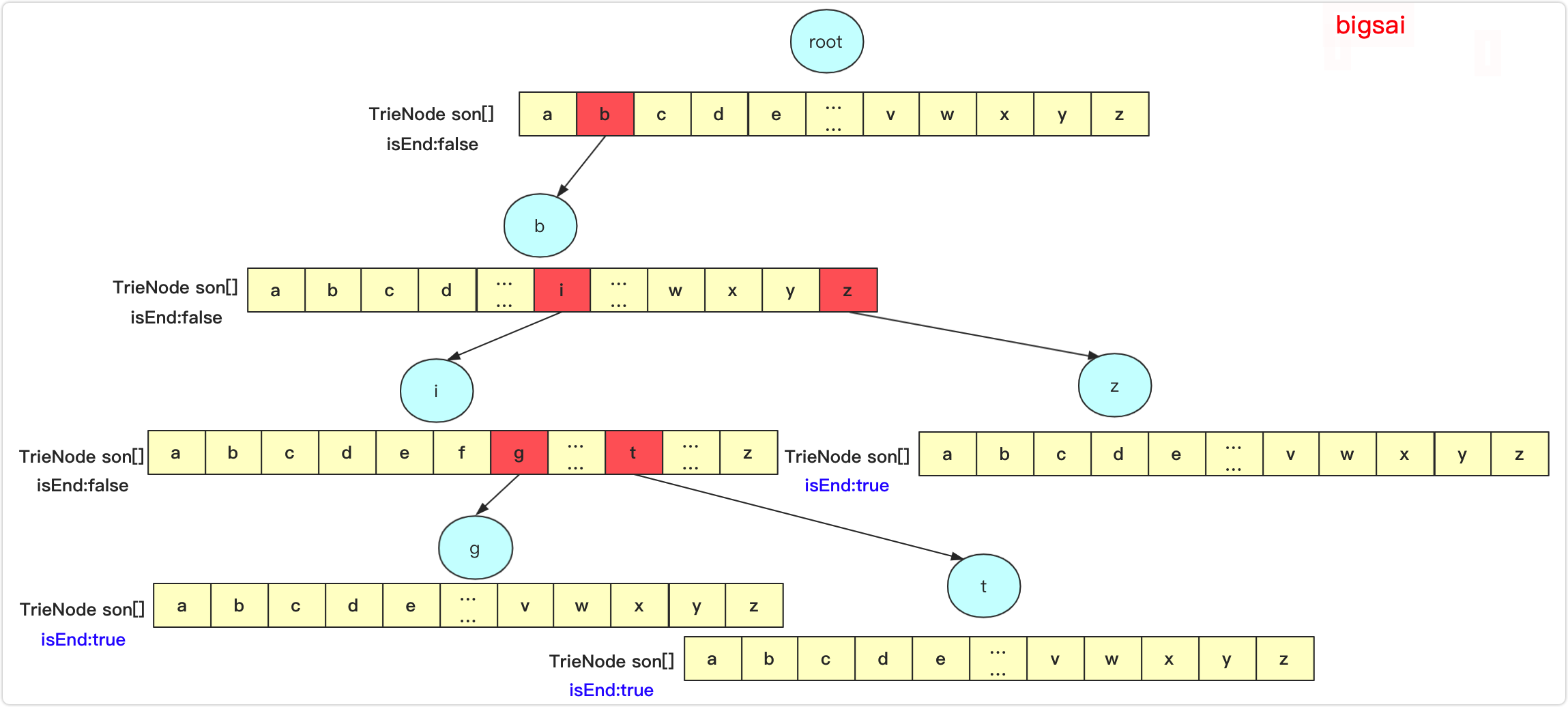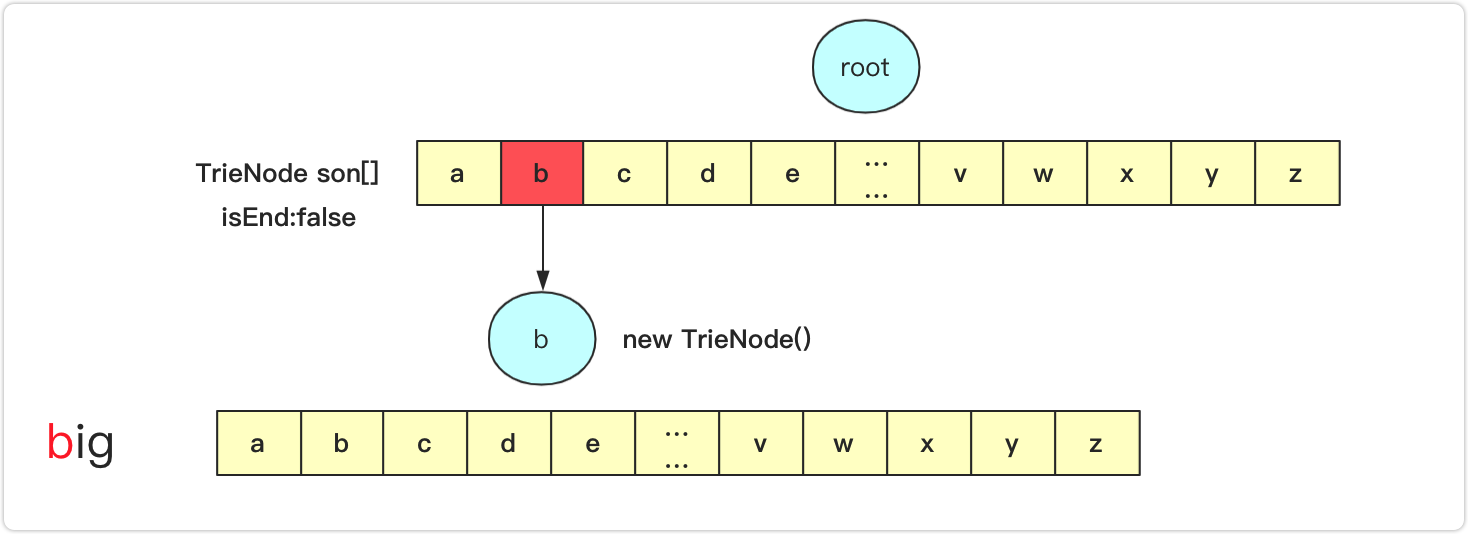```java TrieNode root; /* 初始化 / public Trie() { root=new TrieNode(); }

/* Inserts a word into the trie. / public void insert(String word) { TrieNode node=root;//臨時節點用來列舉 for(int i=0;i<word.length();i++)//列舉字串 { int index=word.charAt(i)-'a';//找到26個對應位置 if(node.son[index]==null)//如果為空需要建立 { node.son[index]=new TrieNode(); } node=node.son[index]; } node.isEnd=true;//最後一個節點 } ```

```java public boolean search(String word) { TrieNode node=root; for(int i=0;i<word.length();i++) { int index=word.charAt(i)-'a'; if(node.son[index]==null)//為null直接返回false { return false; } node=node.son[index]; } return node.isEnd==true; }```

```java public boolean startsWith(String prefix) { TrieNode node=root; for(int i=0;i<prefix.length();i++) { int index=prefix.charAt(i)-'a'; if(node.son[index]==null) { return false; } node=node.son[index]; } //能執行到最後即返回true return true; }```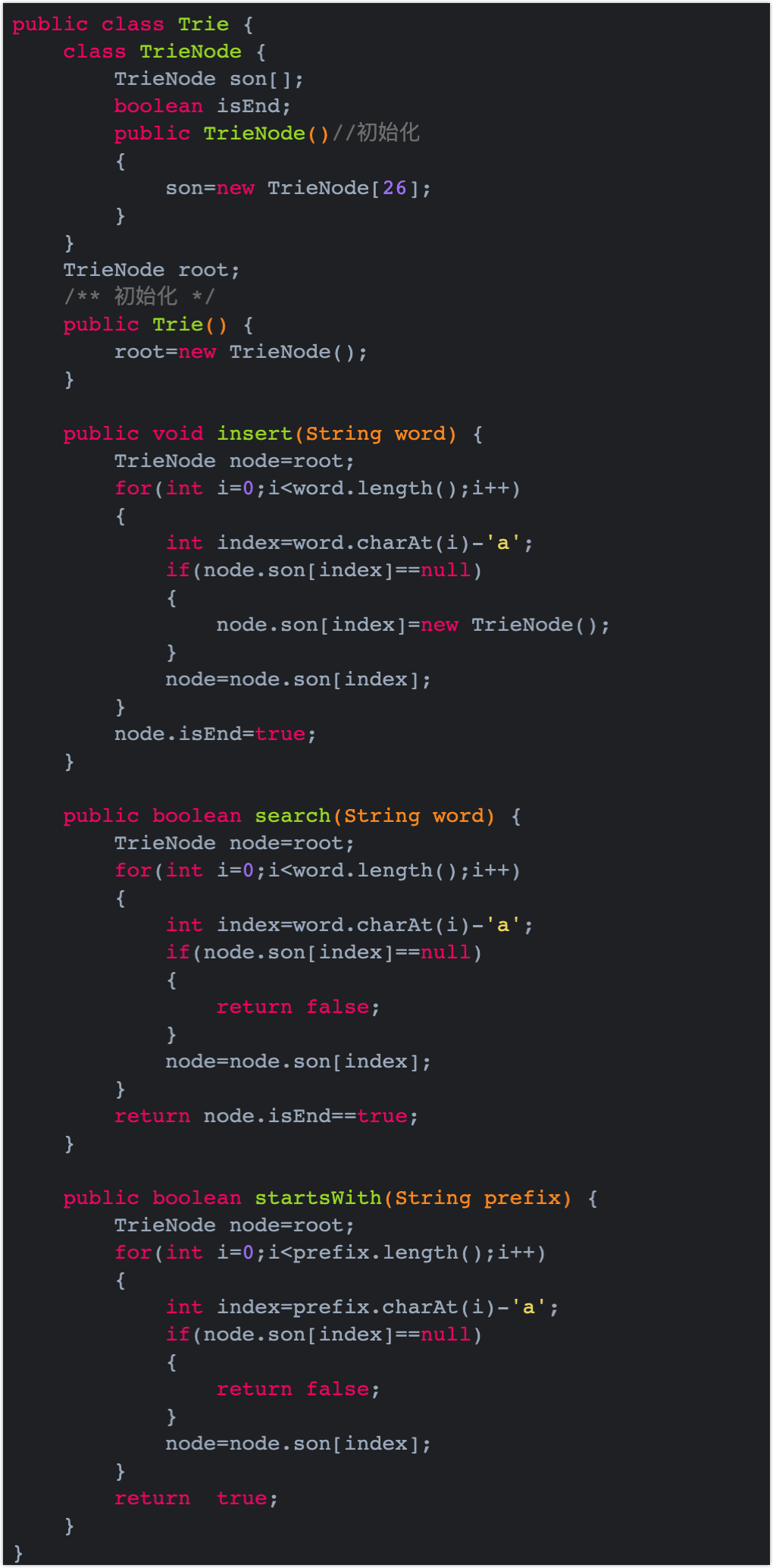### 字典樹小思考

`Map<Character,TrieNode> sonMap;`

```java import java.util.HashMap; import java.util.Map;

public class Trie{ class TrieNode{ Map sonMap; boolean idEnd; public TrieNode() { sonMap=new HashMap<>(); } } TrieNode root; public Trie() { root=new TrieNode(); }

``````public void insert(String word) {
TrieNode node=root;
for(int i=0;i<word.length();i++)
{
char ch=word.charAt(i);
if(!node.sonMap.containsKey(ch))//不存在插入
{
node.sonMap.put(ch,new TrieNode());
}
node=node.sonMap.get(ch);
}
node.idEnd=true;
}

public boolean search(String word) {
TrieNode node=root;
for(int i=0;i<word.length();i++)
{
char ch=word.charAt(i);
if(!node.sonMap.containsKey(ch))
{
return false;
}
node=node.sonMap.get(ch);
}
return node.idEnd==true;//必須標記為true證明有該字串
}

public boolean startsWith(String prefix) {
TrieNode node=root;
for(int i=0;i<prefix.length();i++)
{
char ch=prefix.charAt(i);
if(!node.sonMap.containsKey(ch))
{
return false;
}
node=node.sonMap.get(ch);
}
return true;//執行到最後一步即可
}
``````

} ```

「其他文章」